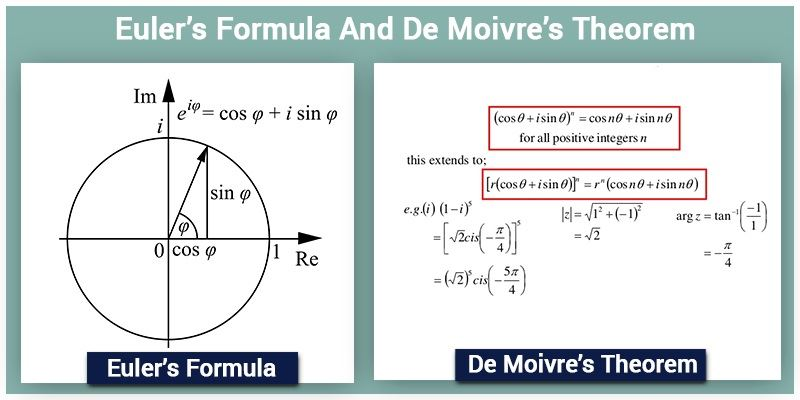# Euler's Formula And De Moivre's Theorem

## Euler’s formula

Euler’s formula states that ‘For any real number $$x$$, $$e^{ix}$$ = $$cos~x~+~i ~sin~x$$.

Let z be a non zero complex number; we can write $$z$$ in the polar form as,

$$z$$ = $$r(cos~θ~+~i~ sin~θ)$$ = $$r~e^{iθ}$$, where $$r$$ is the modulus and $$θ$$ is argument of $$z$$.

Multiplying a complex number $$z$$ with $$e^{iα}$$ gives, $$ze^{iα}$$ = $$re^{iθ}~×~e^{iα}$$ = $$re^{i(α~+~θ)}$$The resulting complex number $$re^{i(α+θ)}$$ will have the same modulus $$r$$ and argument $$(α+θ)$$.## De Moivre’s theorem

It states that for any integer $$n$$,

$$(cos ~θ~+~i ~sin~ θ)^n$$ = $$cos~ (nθ)~+~i ~sin~ (nθ)$$

This can be easily proved using Euler’s formula as shown below.

We know that, $$(cos~ θ~+~i ~sin ~θ)$$ = $$e^{iθ}$$

$$(cos~ θ~+~i ~sin~ θ)^n$$ = $$e^{i(nθ)}$$

Therefore,

$$e^{i(nθ)}$$ = $$cos ~(nθ)~+~i~ sin~ (nθ)$$

$$n^{th}$$ roots of unity

If any complex number satisfies the equation $$z^n$$ = $$1$$, it is known as $$n^{th}$$ root of unity.

Fundamental theorem of algebra says that, an equation of degree $$n$$ will have $$n$$ roots. Therefore, there are $$n$$ values of $$z$$ which satisfies $$z^n$$ = $$1$$.

To find the values of $$z$$, we can write,

$$1$$ = $$cos ~(2kπ)~ + ~i ~sin~ (2kπ)$$, —(1) where k can be any integer.

We have,

$$z^n$$ = $$1$$

$$z$$ = $$1^\frac{1}{n}$$

From (1),

$$z$$ = $$[cos~ (2kπ)~+~i~ sin~ (2kπ)]^{\frac{1}{n}}$$

By De Moivre’s theorem,

$$z$$ = $$[cos~ \left(\frac{2kπ}{n}\right)~+~i ~sin~ \left(\frac{2kπ}{n}\right)]$$, where $$k$$ = $$0, 1, 2, 3, …….., n-1$$

For example; if $$n$$ = $$3$$, then $$k$$ = $$0, 1, 2$$

We know that, $$z$$ = $$cos~ \left(\frac{2kπ}{n}\right)~+~i ~sin~ \left(\frac{2kπ}{n}\right)$$ = $$e^{\frac{2kπi}{n}}$$

Let $$ω$$ = $$cos~ \left(\frac{2π}{n}\right)~+i~ sin ~\left(\frac{2π}{n}\right)$$ = $$e^{\frac{2πi}{n}}$$

$$n^{th}$$ roots of unity are found by,

When $$k$$ = $$0$$; $$z$$ = $$1$$

$$k$$ = $$1$$; $$z$$ = $$ω$$

$$k$$ = $$2$$; $$z$$ = $$ω^2$$

$$k$$ = $$n$$; $$z$$ = $$ω^{n~-~1}$$

Therefore, $$n^{th}$$ roots of unity are $$1, ω, ω^2, ω^3,…….,ω^{n~-~1}$$

• Sum of $$n^{th}$$ roots of unity is,$$1~+~ω~+~ω^2~+~ω^3~+~⋯~+~ω^{n~-~1}$$It is geometric series having first term 1 and common ratio $$ω$$.By using sum of $$n$$ terms of a G.P,$$1~+~ω~+~ω^2~+~ω^3~+~⋯~+~ω^{n~-~1}$$ = $$\frac{1~-~ω^n}{1~-~ω}$$Since $$ω$$ is $$n^{th}$$ root of unity, $$ω^n$$ = $$1$$Therefore, $$1~+~ω~+~ω^2~+~ω^3~+~⋯~+~ω^{n~-~1}$$ = $$0$$

Cube roots of unity:

We know that $$n^{th}$$ roots of unity are $$1, ω, ω^2, ω^3,…….,ω^{n~-~1}$$.

Therefore, cube roots of unity are $$1, ω, ω^2$$ where,

$$ω$$ = $$cos ~\left(\frac{2π}{3}\right)~+~i~ sin~ \left(\frac{2π}{3}\right)$$ = $$\frac{-1~+~√3~ i}{2}$$

$$ω^2$$ = $$cos \left(\frac{4π}{3}\right)~+~i~ sin~ \left(\frac{4π}{3}\right)$$ = $$\frac{-1~-~√3~ i}{2}$$

Sum of the cube roots of the unity,

$$1~+~ω~+~ω^2$$ = $$0$$

Product of cube roots of the unity,

$$1~×~ω~×~ω^2$$ = $$ω^3$$ = $$1$$

Example: $$a$$ and $$b$$ are the roots of the equation $$x^2~+~x~+~1$$ = $$0$$, Find the value of $$a^{17}~+~b^{20}$$

Roots of the equation are

$$a$$ = $$\frac{-1~+~√({1~-~4})}{2}$$ = $$\frac{-1~+~√{3i}}{2}$$

$$b$$ = $$\frac{-1~-~√3~i}{2}$$

Values of $$a$$ and $$b$$ are equal to $$ω$$ and $$ω^2$$ respectively.

$$a^{17}~+~b^{20}$$ = $$ω^{17}~+~(ω^2)^{20}$$ = $$ω^{17}~+~ω^{40}$$ = $$ω^2~+~ω$$

[Since $$ω^{17}$$ = $$ω^{15}~×~ω^2$$ and $$ω^{40}$$ = $$ω^{39}~×~ω$$] [And, since $$1~+~ω~+~ω^2$$ = $$0$$]

Therefore,

$$a^{17}~+~b^{20}$$ = $$-1$$

To know more about complex numbers and its properties, keep learning with BYJU’S and download its app to learn things easily.